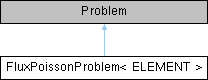FluxPoissonProblem< ELEMENT > Class Template Reference

2D Poisson problem on rectangular domain, discretised with 2D QPoisson elements. Flux boundary conditions are applied along boundary 1 (the boundary where x=L). The specific type of element is specified via the template parameter. More...

Inheritance diagram for FluxPoissonProblem< ELEMENT >:## Public Member Functions

FluxPoissonProblem (PoissonEquations< 2 >::PoissonSourceFctPt source_fct_pt)
Constructor: Pass pointer to source function. More...

~FluxPoissonProblem ()
Destructor (empty) More...

void doc_solution (DocInfo &doc_info)
Doc the solution. DocInfo object stores flags/labels for where the output gets written to. More...

## Private Member Functions

void actions_before_newton_solve ()
Update the problem specs before solve: Reset boundary conditions to the values from the exact solution. More...

void actions_after_newton_solve ()
Update the problem specs after solve (empty) More...

void create_flux_elements (const unsigned &b)
Create Poisson flux elements on the b-th boundary of the problem's mesh. More...

## Private Attributes

unsigned Npoisson_elements
Number of Poisson "bulk" elements (We're attaching the flux elements to the bulk mesh --> only the first Npoisson_elements elements in the mesh are bulk elements!) More...

PoissonEquations< 2 >::PoissonSourceFctPt Source_fct_pt
Pointer to source function. More...

## Detailed Description

template<class ELEMENT>
class FluxPoissonProblem< ELEMENT >

2D Poisson problem on rectangular domain, discretised with 2D QPoisson elements. Flux boundary conditions are applied along boundary 1 (the boundary where x=L). The specific type of element is specified via the template parameter.

Definition at line 93 of file two_d_poisson_flux_bc.cc.

## ◆ FluxPoissonProblem()

template<class ELEMENT >
 FluxPoissonProblem< ELEMENT >::FluxPoissonProblem ( PoissonEquations< 2 >::PoissonSourceFctPt source_fct_pt )

Constructor: Pass pointer to source function.

Constructor for Poisson problem: Pass pointer to source function.

Definition at line 137 of file two_d_poisson_flux_bc.cc.

## ◆ ~FluxPoissonProblem()

template<class ELEMENT >
 FluxPoissonProblem< ELEMENT >::~FluxPoissonProblem ( )
inline

Destructor (empty)

Definition at line 102 of file two_d_poisson_flux_bc.cc.

## ◆ actions_after_newton_solve()

template<class ELEMENT >
 void FluxPoissonProblem< ELEMENT >::actions_after_newton_solve ( )
inlineprivate

Update the problem specs after solve (empty)

Definition at line 115 of file two_d_poisson_flux_bc.cc.

## ◆ actions_before_newton_solve()

template<class ELEMENT >
 void FluxPoissonProblem< ELEMENT >::actions_before_newton_solve
private

Update the problem specs before solve: Reset boundary conditions to the values from the exact solution.

Definition at line 254 of file two_d_poisson_flux_bc.cc.

References TanhSolnForPoisson::get_exact_u().

## ◆ create_flux_elements()

template<class ELEMENT >
 void FluxPoissonProblem< ELEMENT >::create_flux_elements ( const unsigned & b )
private

Create Poisson flux elements on the b-th boundary of the problem's mesh.

Create Poisson Flux Elements on the b-th boundary of the Mesh.

Definition at line 221 of file two_d_poisson_flux_bc.cc.

Referenced by FluxPoissonProblem< ELEMENT >::FluxPoissonProblem().

## ◆ doc_solution()

template<class ELEMENT >
 void FluxPoissonProblem< ELEMENT >::doc_solution ( DocInfo & doc_info )

Doc the solution. DocInfo object stores flags/labels for where the output gets written to.

Doc the solution: doc_info contains labels/output directory etc.

Definition at line 296 of file two_d_poisson_flux_bc.cc.

References TanhSolnForPoisson::get_exact_u().

Referenced by main().

## ◆ Npoisson_elements

template<class ELEMENT >
 unsigned FluxPoissonProblem< ELEMENT >::Npoisson_elements
private

Number of Poisson "bulk" elements (We're attaching the flux elements to the bulk mesh --> only the first Npoisson_elements elements in the mesh are bulk elements!)

Definition at line 124 of file two_d_poisson_flux_bc.cc.

Referenced by FluxPoissonProblem< ELEMENT >::FluxPoissonProblem().

## ◆ Source_fct_pt

template<class ELEMENT >
 PoissonEquations<2>::PoissonSourceFctPt FluxPoissonProblem< ELEMENT >::Source_fct_pt
private

Pointer to source function.

Definition at line 127 of file two_d_poisson_flux_bc.cc.

Referenced by FluxPoissonProblem< ELEMENT >::FluxPoissonProblem().

The documentation for this class was generated from the following file: